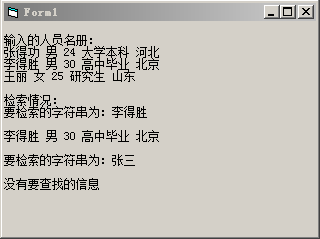﻿ VB编程：有如下人员名册：姓名 性别 年龄 文件程序 籍贯 张得功 男 24 大学本科 河北 试编写一个程序，对该名册进行检索。 - VB程序设计教程答案

VB编程：有如下人员名册：姓名 性别 年龄 文件程序 籍贯 张得功 男 24 大学本科 河北 试编写一个程序，对该名册进行检索。

2016-07-14 10:24:29  分类: VB程序设计教程第4版答案刘炳文  参与: AddViewCount(27)人

……

张得功   男   24   大学本科   河北

（1）使用动态数组，输入的人数可以根据实际情况改变。
（2）当检索名册中不存在的人名时，输出相应的信息。
（3）每次检索结束后，询问是否继续检索，根据输入的信息确定是否结束程序。

Option Base 1 '要想数组下标不是从O开始，而是从1开始，就要加上这句

Private Sub Form_Click()

Dim arr(), s
s = Array("姓名", "性别", "年龄", "文化程度", "籍贯")
n = InputBox("请输入人数")
n = Val(n)
ReDim arr(n, 5)
Print
For i = 1 To n
For j = 1 To 5
arr(i, j) = InputBox("请输入第" & i & " 个人的" & s(j))
Next j
Next i

Print "输入的人员名册："
For i = 1 To n
For j = 1 To 5
Print arr(i, j); " ";
Next j
Print
Next i

Print
Print "检索情况："
Do
sn = InputBox("请输入要查找的名字")
For i = 1 To n
For j = 1 To 5
If sn = arr(i, 1) Then
row = i
Exit For
Else
row = 0
End If
Next j
If row <> 0 Then Exit For
Next i

Print "要检索的字符串为："; sn
Print
If row <> 0 Then
For i = 1 To 5
Print arr(row, i); " ";
Next i
Print
Else
Print "没有要查找的信息"
End If
Print
a = MsgBox("是否继续检索？", 19, "选择")
If a <> 6 Then
Exit Do
End If
Loop

End Subvisual basic 程序设计教程第四版答案 刘炳文 编著
Visual Basic 程序设计教程题解与上机指导 第四版 刘炳文

<< 上一篇下一篇 >>